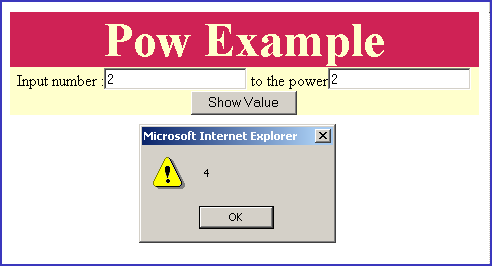Tutorials

JavaScript pow() method

Some times programmers may need to evaluate the value by the power of some numbers therefore JavaScript provides an in-built function Math.pow().

Some times programmers may need to evaluate the value by the power of some numbers therefore JavaScript provides an in-built function Math.pow().

JavaScript pow() method

Some times programmers may need to evaluate the value by the power of some numbers therefore JavaScript provides an in-built function Math.pow().

Syntax:

 Math.pow(x,y);

Math.pow(x,y) returns value of x to the power of y (xy). Where x and y both are required.

In the following example we will describe you the use of Math.pow() method with very simple code. Here in this example code we have created two input boxes into which we will take the values of x and y from the user side.

Description of code:

Take two values x and y from the user and then apply the Math object's method pow() onto it. When user clicks on the button "Show Value" it calls the method callPow() as defined in the JavaScript within our <script></script> tag.

 function callPow(){ var val= document.getElementById("txt").value; var power= document.getElementById("txt2").value; alert(Math.pow(val,power)); }

Here we have taken the values of x and y into the "val" and "power" variables. Full html code for this example is as follows :


Pow Example
Input number : to the power

Output :

Input numbers into the text boxes :then click on button "Show Value" to view results.Calculate 3 to the power 5 (35).